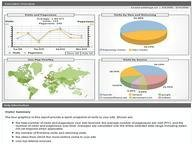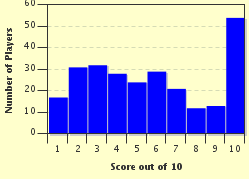FREE! Click here to Join FunTrivia. Thousands of games, quizzes, and lots more!# Theoretical Basic Probability Trivia Quiz

### This is a quiz on the language and basic results of probability that would be found in a course in finite mathematics. Good luck!

A multiple-choice quiz by rodney_indy. Estimated time: 5 mins.

Author
rodney_indy
Time
5 mins
Type
Multiple Choice
Quiz #
269,802
Updated
Jul 23 22
# Qns
10
Difficulty
Tough
Avg Score
5 / 10
Plays
1157
This quiz has 2 formats: you can play it as a or as shown below.
Scroll down to the bottom for the answer key.
1. Suppose E and F are mutually exclusive events in a sample space S with probabilities .4 and .3 respectively. What is the probability of their union? Hint

0.7
0.4
0.1
0.3

#### NEXT>

2. Suppose E is an event in a sample space S with probability .3. What is the probability of the complement of E? Hint

0.3
1
0
0.7

#### NEXT>

3. Suppose E and F are events in a sample space S. Suppose further that E has probability .2, F has probability .6, and the intersection of E and F has probability .1. What is the probability of the union of E and F? Hint

0.68
0.6
0.7
0.8

#### NEXT>

4. Suppose E and F are independent events in a sample space S. Suppose further that E has probability .3 and F has probability .4. What is the probability of the intersection of E and F? Hint

1
0.7
0.12
0

#### NEXT>

5. Suppose E and F are independent events in a sample space S. Suppose further that E has probability .3 and F has probability .4. What is the probability of the union of E and F? Hint

0.12
0.7
0.58
0.4

#### NEXT>

6. Suppose E and F are mutually exclusive events in a sample space S. Suppose further that E has probability .3 and F has probability .4. What is the probability of the intersection of E and F? Hint

0.12
0.7
0
0.3

#### NEXT>

7. Suppose E and F are events in a sample space S. Suppose further that E has probability .3, F has probability .4, and the intersection of E and F has probability .2. Find the probability of the intersection of E and (the complement of F). Hint

0
0.3
0.2
0.1

#### NEXT>

8. Suppose E and F are events in a sample space S. Suppose further that E has probability .5, F has probability .6, and the intersection of E and F has probability .2. Find the probability of the union of E and (the complement of F). Hint

0.3
0.6
0.5
0.8

#### NEXT>

9. Suppose E and F are events in a sample space S. Suppose further that E has probability .8 and F has probability .9. What is the largest possible value for the probability of the intersection of E and F? Hint

0.9
0.7
0.8
0.2

#### NEXT>

10. Suppose E and F are events in a sample space S. Suppose further that E has probability .8 and F has probability .9. What is the smallest possible value for the probability of the intersection of E and F? Hint

0.9
0.7
0.8
0.1

 (Optional) Create a Free FunTrivia ID to save the points you are about to earn:Select a User ID:Choose a Password:Your Email:

Most Recent Scores
Sep 13 2023 : Guest 136: 0/10
Aug 23 2023 : Guest 105: 8/10

Score DistributionQuiz Answer Key and Fun Facts
1. Suppose E and F are mutually exclusive events in a sample space S with probabilities .4 and .3 respectively. What is the probability of their union?

Mutually exclusive events are events whose intersection is the empty set. So the probability of their union is just the sum of their probabilities, which is .7.
2. Suppose E is an event in a sample space S with probability .3. What is the probability of the complement of E?

The probability of an event and its complement must sum to 1, since they are mutually exclusive and their union is the entire sample space S. So the probability of the complement of E is 1 - .3 = .7.
3. Suppose E and F are events in a sample space S. Suppose further that E has probability .2, F has probability .6, and the intersection of E and F has probability .1. What is the probability of the union of E and F?

For any two sets, the probability of their union is just the sum of their probabilities, minus the probability of their intersection. This is called the principle of inclusion/exclusion. The reason you have to subtract the probability of the intersection is because the intersection of the two events is itself a subset of each event, so its probability gets counted twice. So the probability we're after is just .2 + .6 - .1 = .7.
4. Suppose E and F are independent events in a sample space S. Suppose further that E has probability .3 and F has probability .4. What is the probability of the intersection of E and F?

If E and F are independent events, the probability of their intersection is found by multiplying their respective probabilities. So the answer in this case is .3 * .4 = .12.
5. Suppose E and F are independent events in a sample space S. Suppose further that E has probability .3 and F has probability .4. What is the probability of the union of E and F?

By the principle of inclusion/exclusion, the probability of the union of E and F is the probability of E plus the probability of F minus the probability of the intersection of E and F. But E and F are independent, so the probability of their intersection is just .3 * .4 = .12. Hence the probability of their union is .3 + .4 - .12 = .58.
6. Suppose E and F are mutually exclusive events in a sample space S. Suppose further that E has probability .3 and F has probability .4. What is the probability of the intersection of E and F?

Recall that mutually exclusive events are events whose intersection is the empty set. The empty set has probability 0.
7. Suppose E and F are events in a sample space S. Suppose further that E has probability .3, F has probability .4, and the intersection of E and F has probability .2. Find the probability of the intersection of E and (the complement of F).

Note that E is the disjoint union of (E intersect (the complement of F)) and (E intersect F). Since the probability of E is .3 and the probability of (E intersect F) is .2, the probability we want is just .3 - .2 = .1.
8. Suppose E and F are events in a sample space S. Suppose further that E has probability .5, F has probability .6, and the intersection of E and F has probability .2. Find the probability of the union of E and (the complement of F).

The union of E and (the complement of F) can be written as the disjoint union of E and ((the complement of E) intersect (the complement of F)). By the principle of inclusion/exclusion, the probability of the union of E and F is just the probability of E plus the probability of F minus the probability of the intersection of E and F. So the probability of the union of E and F is .5 + .6 - .2 = .9. Now by one of DeMorgan's laws, the complement of the union of E and F is the intersection of their complements, thus the probability of ((the complement of E) intersect (the complement of F)) is just 1 - .9 = .1. So the probability we want is (from the first sentence) .5 + .1 = .6. Note that this problem could also be solved by drawing a Venn diagram.
9. Suppose E and F are events in a sample space S. Suppose further that E has probability .8 and F has probability .9. What is the largest possible value for the probability of the intersection of E and F?

The intersection of E and F is a subset of both E and F, hence its probability must be less than or equal to each of these probabilities. So the largest it can be is the smallest of the probabilities of E or F, which is .8.
10. Suppose E and F are events in a sample space S. Suppose further that E has probability .8 and F has probability .9. What is the smallest possible value for the probability of the intersection of E and F?